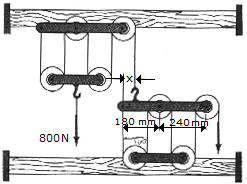# Engineering Mechanics - Structural Analysis - Discussion

### Discussion :: Structural Analysis - General Questions (Q.No.2)

2.The principles of a differential chain block are indicated schematically in the figure. Determine the magnitude of force P needed to support the 800-N force. Also compute the distance x where the cable must be attached to bar AB so the bar remains horizontal. All pulleys have a radius of 60 mm.

 [A]. P = 80 N, x = 240 mm [B]. P = 80 N, x = 180 mm [C]. P = 40 N, x = 180 mm [D]. P = 40 N, x = 240 mm

Explanation:

No answer description available for this question.

 Student said: (Aug 28, 2012) Please explain this problem

 Ameet Kumar said: (Apr 26, 2016) Please explain this question and solution.

 Ismayil said: (Mar 6, 2017) At Left block. Each rope on left block 800/4=200N. Last rope also is equal 200N. At Right block: Same principle, P=200/5=40N. Let's call left block top line joint points A, B(hook point), C, D. B=200. Each Pulley point load is 40+40=80N. C=80. D=80. sum of M(A)=0. 200 * x - 180 * 80 - 420 * 80 = 0. 200 * x = 48000. x = 240mm. Good luck.